# Parabola 3

Find the equation of a parabola with its focus at (0,2) and its vertex at the origin.
$f:y={x}^{2}+bx+c$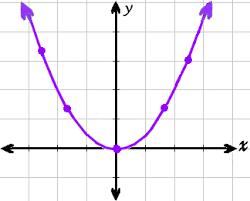Did you find an error or inaccuracy? Feel free to write us. Thank you!Tips to related online calculators
Do you have a linear equation or system of equations and looking for its solution? Or do you have a quadratic equation?

#### You need to know the following knowledge to solve this word math problem:

We encourage you to watch this tutorial video on this math problem:

## Related math problems and questions:

• Sphere from tree pointsEquation of sphere with three point (a,0,0), (0, a,0), (0,0, a) and center lies on plane x+y+z=a
• Intersections 3Find the intersections of the circles x2 + y2 + 6 x - 10 y + 9 = 0 and x2 + y2 + 18 x + 4 y + 21 = 0
• Non linear eqsSolve the system of non-linear equations: 3x2-3x-y=-2 -6x2-x-y=-7Quadratic equation 7x2+bx+c=0 has roots x1 = 67 and x2 = -84. Calculate the coefficients b and c.
• ParabolaFind the equation of a parabola that contains the points at A[6; -5], B[14; 9], C[23; 6]. (use y = ax2+bx+c)
• Linear systemSolve a set of two equations of two unknowns: 1.5x+1.2y=0.6 0.8x-0.2y=2
• Function 3Function f(x)=a(x-r)(x-s) the graph of the function has x- intercept at (-4, 0) and (2, 0) and passes through the point (-2,-8). Find constant a, r, s.
• Roots count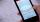Substitute the numbers/0,1,2,3/into the equation as x: (x - 1) (x - 3) (x + 1) = 0 Which of them is its solution? Is there another number that solves this equation?
• Roots and coefficient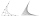In the equation 2x ^ 2 + bx-9 = 0 is one root x1 = -3/2. Determine the second root and the coefficient b.
• ProveProve that k1 and k2 are the equations of two circles. Find the equation of the line that passes through the centers of these circles. k1: x2+y2+2x+4y+1=0 k2: x2+y2-8x+6y+9=0
• CenterCalculate the coordinates of the circle center: x2 -4x + y2 +10y +25 = 0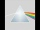Please determine the solvability conditions of the equation, solve the equation and perform the test: x divided by x squared minus 2x plus1 the whole minus x + 3 divided by x squared minus 1 this is equal to 0: x/(x2-2x+1) - (x+3)/( x2-1) = 0Solve quadratic equation: 2x²- 2(x-7)²+5x=0Ellipse is expressed by equation 9x2 + 25y2 - 54x - 100y - 44 = 0. Find the length of primary and secondary axes, eccentricity, and coordinates of the center of the ellipse.If the square root of 3m2 +22 and -x = 0, and x=7, what is m?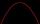Solve pure quadratic equation -5x2 +10 = 0.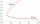If x=4 and y=-3, what is the value of the expression x² + 2xy - 4x + 3y + y²?